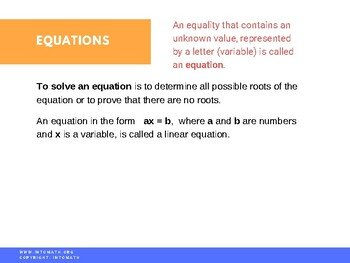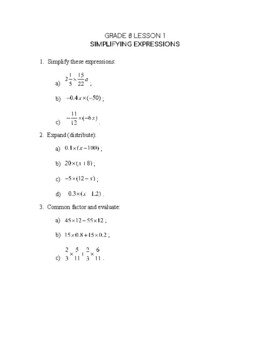CA\$2.00

In this lesson you will learn how to solve one step and multi step linear equations.

For example, x – 3 = 10  or  2h + 1 = – 9.

The lesson demonstrates how to solve linear equations with brackets.

For example, 2(x – 1) = 16.

Such equations are solved using inverse operations and reverse BEDMAS. The first step is always expanding the expression with brackets using the distributive multiplication property.

You will also discover the Pythagorean Theorem.

You will learn when and why to use it, how to set it up and solve the equation.